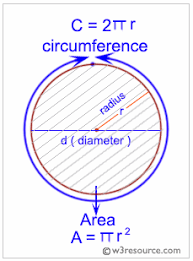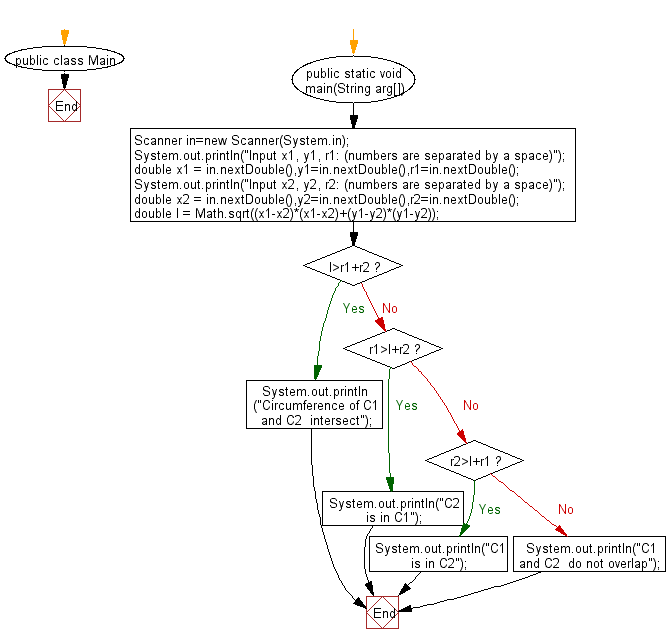# Java Exercises: Test if circumference of two circles intersect or overlap

## Java Basic: Exercise-224 with Solution

There are two circles C1 with radius r1, central coordinate (x1, y1) and C2 with radius r2 and central coordinate (x2, y2)
Write a Java program to test the followings -
"C2 is in C1" if C2 is in C1
"C1 is in C2" if C1 is in C2
"Circumference of C1 and C2 intersect" if circumference of C1 and C2 intersect, and
"C1 and C2 do not overlap" if C1 and C2 do not overlap.

Input:
Input numbers (real numbers) are separated by a space.

Pictorial Presentation:Sample Solution:

Java Code:

`````` import java.util.Scanner;
public class Main
{
public static void main(String arg[])
{
Scanner in=new Scanner(System.in);
System.out.println("Input x1, y1, r1: (numbers are separated by a space)");
double x1 = in.nextDouble(),y1=in.nextDouble(),r1=in.nextDouble();
System.out.println("Input x2, y2, r2: (numbers are separated by a space)");
double x2 = in.nextDouble(),y2=in.nextDouble(),r2=in.nextDouble();
double l = Math.sqrt((x1-x2)*(x1-x2)+(y1-y2)*(y1-y2));
if(l>r1+r2)
System.out.println("Circumference of C1  and C2  intersect");
else if(r1>l+r2)
System.out.println("C2  is in C1");
else if(r2>l+r1)
System.out.println("C1  is in C2");
else
System.out.println("C1 and C2  do not overlap");
}
}
```
```

Sample Output:

```Input x1, y1, r1: (numbers are separated by a space)
5 6 8 7
Input x2, y2, r2: (numbers are separated by a space)
8 9 5 4
C1 and C2  do not overlap
```

Flowchart:Java Code Editor:

What is the difficulty level of this exercise?

﻿

## Java: Tips of the Day

Parsing dates:

```import java.io.*;
import java.util.*;
import java.text.*;

String s = "2001/09/23 14:39";

SimpleDateFormat formatter = new SimpleDateFormat ("yyyy/MM/dd H:mm");
Date d = formatter.parse(s, new ParsePosition(0));
```